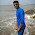# MATLAB Program for Formation of Bus Impedance Matrices | EE1404 – Power System Simulation Laboratory

AIM:
To determine the bus impedance matrices for the given power system network.

SOFTWARE REQUIRED:
MATLAB 5.3

THEORY:
FORMATION OF Z-BUS MATRIX
In bus impedance matrix the elements on the main diagonal are called driving point
impedance and the off-diagonal elements are called the transfer impedance of the buses or nodes.
The bus impedance matrix are very useful in fault analysis.
The bus impedance matrix can be determined by two methods. In one method we can form
the bus admittance matrix and than taking its inverse to get the bus impedance matrix. In another
method the bus impedance matrix can be
directly formed from the reactance diagram and this
method requires the knowledge of the modifications of existing bus impedance matrix due to
addition of new bus or addition of a new line (or impedance) between existing buses.

PROCEDURE:
1. Enter the command window of the MATLAB.
2. Create a new M – file by selecting File - New – M – File
3. Type and save the program in the editor Window
4. Execute the program by either pressing Tools – Run.
5. View the results.

EXERCISE:
(i) Determine the and Z bus matrix for the power system network shown in fig.
(ii) Check the results obtained in using MATLAB.

PROGRAM:
z = [0 1 0 1.0
0 2 0 0.8
1 2 0 0.4
1 3 0 0.2
2 3 0 0.2
3 4 0 0.08];
Y = ybus(z)
Ibus = [-j*1.1; -j*1.25; 0; 0];
Zbus = inv(Y)
Vbus = Zbus*Ibus

RESULT:
Thus the bus impedance matrices for the given power system network determined.
Previous
Next Post »

Mannan
AUTHORMannan
AUTHOR

I'm urgent, it does not worked for me